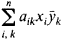# Hermitian Matrix

(redirected from Hermitian matrices)
Also found in: Dictionary.
Related to Hermitian matrices: Unitary matrices

## Hermitian matrix

[er′mish·ən ′mā·triks]
(mathematics)
A matrix which equals its conjugate transpose matrix, that is, is self-adjoint.
McGraw-Hill Dictionary of Scientific & Technical Terms, 6E, Copyright © 2003 by The McGraw-Hill Companies, Inc.
The following article is from The Great Soviet Encyclopedia (1979). It might be outdated or ideologically biased.

## Hermitian Matrix

(or self-adjoint matrix), a matrix coincident with its adjoint, that is, a matrix such that aik= āki, where ā is the complex conjugate of the number a. If the elements of a Hermitian matrix are real, then the matrix is symmetric. A Hermitian matrix has real eigenvalues λ1, λ2, …, λn and corresponds to a linear transformation in a complex n-dimensional space that reduces to stretchings by ǀλiǀ in n mutually perpendicular directions and reflections in the planes orthogonal to the directions for which λi < 0. A bilinear formwhose coefficients form a Hermitian matrix, is called a Hermitian form. Any matrix can be written in the form A1 + iA2, where A1 and A2 are Hermitian matrices, and in the form A ∪, where A is a Hermitian matrix and U is a unitary matrix. If A and B are Hermitian matrices, then A B is a Hermitian matrix if and only if A and B commute.

References in periodicals archive ?
Thus all Hermitian matrices R, X [member of] [C.sup.nxn] with R positive definite and X positive semidefinite satisfy the inequalities
THEOREM 2.2 For any pair of r x r Hermitian matrices M and N, let us set
Fisk, "A very short proof of Cauchy's interlace theorem for eigenvalues of Hermitian matrices," The American Mathematical Monthly, vol.
The Euclidean Jordan algebra [H.sub.3.sup.8] of 3 x 3 Hermitian matrices over the Octonions is simple with Pierce constant d = 8 but does not admit a representation.
Some symbols and notations: Let [C.sup.mxn.sub.r] be the set of all mxn complex matrices with rank r, H[C.sup.mxn] be the set of all n x n Hermitian matrices. Denoted by [A.sup.+], A*, rank(A) the Moore-Penrose generalized inverse, conjugate transpose, rank of matrix A, respectively.
Here [bar.D] is the diagonal part of A and [bar.H] and [bar.V] are Hermitian matrices. For the solution of (1.1), the variant of Peacemen--Rachford iterative method is considered as
The core of the calculation is a series of matrix diagonalizations carried out by NAG routines designed to handle Hermitian matrices. The least-squares fitting is also performed by NAG routines.
We shall make frequent use of Weyl's theorem [19, Theorem 4.3.1], which says that if A and B are Hermitian matrices, then
The software package handles real and complex matrices and supplies specific routines for symmetric and Hermitian matrices. A distinct attention is given to matrices arising from Markov chains.
Hence H([M.sub.n](C)) is the set of all Hermitian matrices, and H(C(Y)) = [C.sub.R](Y).
The second iteration finds two more eigenvalues and further iterations show smooth convergence reminding of interlacing eigenvalues for Hermitian matrices.
Under the conditions of Theorem 10, the equilibrium point of system (1) is globally [mu]-stable, if there exist six positive definite Hermitian matrices [P.sub.1], [P.sub.2], [P.sub.3], [P.sub.4], [P.sub.5], and [P.sub.6], two real positive diagonal matrices [R.sub.1] and [R.sub.2], three complex matrices [Q.sub.1], [Q.sub.2], and [Q.sub.3], a positive differential function [mu](t), and three constants [alpha] [greater than or equal to] 0, [beta] > 0, and T > 0 such that

Site: Follow: Share:
Open / Close